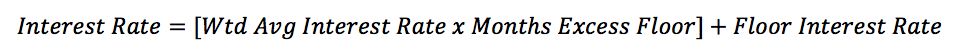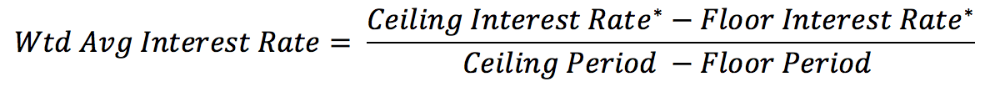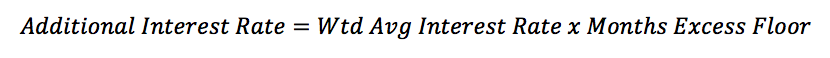# Interest Rate Calculation

The interest rate used for the Black Scholes Model must have a time period that matches the expected term of the option. For example, if the option has an expected term of seven years, the interest rate should be the 7-year US. Treasury Rate.

Carta utilizes the daily U.S. Treasury Rate provided by the  Federal Reserve. Based on the expected term of the life of each individual grant, the associated rate will be applied. Often times the expected term of a grant will not perfectly align with the interest rate period provided by the Federal reserve.

#### In these cases, Carta will compute a new interest rate by using a weighted calculation based on the floor and ceiling rate.

Here is the formula Carta uses to determine the interest rate:The Federal Reserve issues 1, 3, 5, 7, 10, 20, and 30-year interest rates. Carta will allocate the appropriate interest rate to the grant based on the grant’s expected term (See ASC 718 Mark to Market Report(*.xls) tab 'EE Expense 201x,' column 'Current Interest Rate'). If the expected term does not match any of the years listed above, it will fall between a range. The floor would be the lower end of the range whereas the ceiling will be the higher end.

The formula above can be split into two sections: the floor’s base interest rate and the additional interest rate. For example, if the expected life of the grant is 8.5 years, the floor would be 7 years and the ceiling would be 10 years. Since there is no applicable interest rate for an 8.5-year grant, we use the floor’s interest rate and add in an appropriate additional interest rate to account for the 1.5-year difference.

#### To determine the additional interest rate needed, we take the weighted average interest rate and multiply it by the difference between the grant’s expected term and the floor period.

The weighted average interest rate is determined by the following formula:*The rates are based on the data extracted from the Federal Reserve.

#### The additional interest rate then uses the weighted average interest rate as one of its inputs.

The overall additional interest rate is computed with the following formula:We are then able to calculate the appropriate interest rate for the grant by adding the additional interest rate to the floor interest rate.

Was this article useful? Thanks for the feedback There was a problem submitting your feedback. Please try again later.